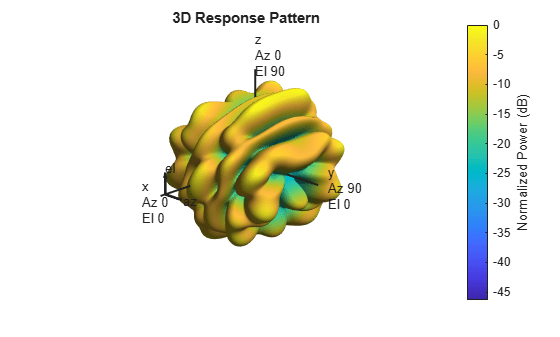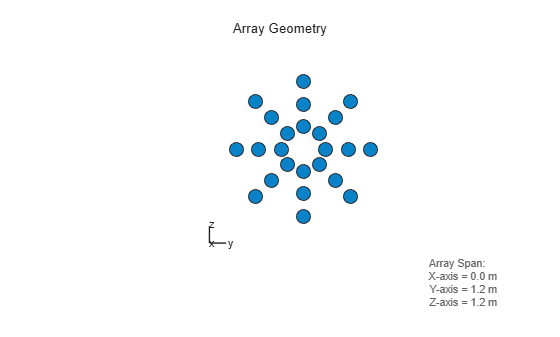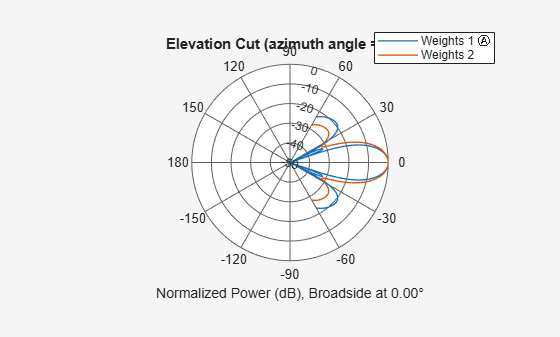pattern

System object: phased.HeterogeneousConformalArray
Package: phased

Plot heterogeneous conformal array pattern

Syntax

pattern(sArray,FREQ)
pattern(sArray,FREQ,AZ)
pattern(sArray,FREQ,AZ,EL)
pattern(___,Name,Value)
[PAT,AZ_ANG,EL_ANG] = pattern(___)

Description

pattern(sArray,FREQ) plots the 3-D array directivity pattern (in dBi) for the array specified in sArray. The operating frequency is specified in FREQ.

The integration used when computing array directivity has a minimum sampling grid of 0.1 degrees. If an array pattern has a beamwidth smaller than this, the directivity value will be inaccurate.

pattern(sArray,FREQ,AZ) plots the array directivity pattern at the specified azimuth angle.

pattern(sArray,FREQ,AZ,EL) plots the array directivity pattern at specified azimuth and elevation angles.

pattern(___,Name,Value) plots the array pattern with additional options specified by one or more Name,Value pair arguments.

[PAT,AZ_ANG,EL_ANG] = pattern(___) returns the array pattern in PAT. The AZ_ANG output contains the coordinate values corresponding to the rows of PAT. The EL_ANG output contains the coordinate values corresponding to the columns of PAT. If the 'CoordinateSystem' parameter is set to 'uv', then AZ_ANG contains the U coordinates of the pattern and EL_ANG contains the V coordinates of the pattern. Otherwise, they are in angular units in degrees. UV units are dimensionless.

Note

This method replaces the plotResponse method. See Convert plotResponse to pattern for guidelines on how to use pattern in place of plotResponse.

Input Arguments

expand all

Heterogeneous conformal array, specified as a phased.HeterogeneousConformalArray System object.

Example: sArray= phased.HeterogeneousConformalArray;

Frequencies for computing directivity and patterns, specified as a positive scalar or 1-by-L real-valued row vector. Frequency units are in hertz.

• For an antenna, microphone, or sonar hydrophone or projector element, FREQ must lie within the range of values specified by the FrequencyRange or FrequencyVector property of the element. Otherwise, the element produces no response and the directivity is returned as –Inf. Most elements use the FrequencyRange property except for phased.CustomAntennaElement and phased.CustomMicrophoneElement, which use the FrequencyVector property.

• For an array of elements, FREQ must lie within the frequency range of the elements that make up the array. Otherwise, the array produces no response and the directivity is returned as –Inf.

Example: [1e8 2e6]

Data Types: double

Azimuth angles for computing directivity and pattern, specified as a 1-by-N real-valued row vector where N is the number of azimuth angles. Angle units are in degrees. Azimuth angles must lie between –180° and 180°.

The azimuth angle is the angle between the x-axis and the projection of the direction vector onto the xy plane. When measured from the x-axis toward the y-axis, this angle is positive.

Example: [-45:2:45]

Data Types: double

Elevation angles for computing directivity and pattern, specified as a 1-by-M real-valued row vector where M is the number of desired elevation directions. Angle units are in degrees. The elevation angle must lie between –90° and 90°.

The elevation angle is the angle between the direction vector and xy-plane. The elevation angle is positive when measured towards the z-axis.

Example: [-75:1:70]

Data Types: double

Name-Value Arguments

Specify optional comma-separated pairs of Name,Value arguments. Name is the argument name and Value is the corresponding value. Name must appear inside quotes. You can specify several name and value pair arguments in any order as Name1,Value1,...,NameN,ValueN.

Plotting coordinate system of the pattern, specified as the comma-separated pair consisting of 'CoordinateSystem' and one of 'polar', 'rectangular', or 'uv'. When 'CoordinateSystem' is set to 'polar' or 'rectangular', the AZ and EL arguments specify the pattern azimuth and elevation, respectively. AZ values must lie between –180° and 180°. EL values must lie between –90° and 90°. If 'CoordinateSystem' is set to 'uv', AZ and EL then specify U and V coordinates, respectively. AZ and EL must lie between -1 and 1.

Example: 'uv'

Data Types: char

Displayed pattern type, specified as the comma-separated pair consisting of 'Type' and one of

• 'directivity' — directivity pattern measured in dBi.

• 'efield' — field pattern of the sensor or array. For acoustic sensors, the displayed pattern is for the scalar sound field.

• 'power' — power pattern of the sensor or array defined as the square of the field pattern.

• 'powerdb' — power pattern converted to dB.

Example: 'powerdb'

Data Types: char

Array orientation, specified as a 3-by-1 real-valued column vector containing the rotation angles with respect to the x-, y-, and z-axes of the local coordinate system, respectively.

Display normalized pattern, specified as the comma-separated pair consisting of 'Normalize' and a Boolean. Set this parameter to true to display a normalized pattern. This parameter does not apply when you set 'Type' to 'directivity'. Directivity patterns are already normalized.

Data Types: logical

View the array geometry along with the 3D radiation pattern, specified as false or true.

Data Types: logical

Show the local coordinate axes, specified as true or false.

Data Types: logical

Show the colorbar, specified as true or false.

Data Types: logical

Handle to the axes along which the array geometry is displayed specified as a scalar.

Plotting style, specified as the comma-separated pair consisting of 'Plotstyle' and either 'overlay' or 'waterfall'. This parameter applies when you specify multiple frequencies in FREQ in 2-D plots. You can draw 2-D plots by setting one of the arguments AZ or EL to a scalar.

Data Types: char

Polarized field component to display, specified as the comma-separated pair consisting of 'Polarization' and 'combined', 'H', or 'V'. This parameter applies only when the sensors are polarization-capable and when the 'Type' parameter is not set to 'directivity'. This table shows the meaning of the display options.

'Polarization'Display
'combined'Combined H and V polarization components
'H'H polarization component
'V'V polarization component

Example: 'V'

Data Types: char

Signal propagation speed, specified as the comma-separated pair consisting of 'PropagationSpeed' and a positive scalar in meters per second.

Example: 'PropagationSpeed',physconst('LightSpeed')

Data Types: double

Array weights, specified as the comma-separated pair consisting of 'Weights' and an N-by-1 complex-valued column vector or N-by-L complex-valued matrix. Array weights are applied to the elements of the array to produce array steering, tapering, or both. The dimension N is the number of elements in the array. The dimension L is the number of frequencies specified by FREQ.

Weights DimensionFREQ DimensionPurpose
N-by-1 complex-valued column vectorScalar or 1-by-L row vectorApplies a set of weights for the single frequency or for all L frequencies.
N-by-L complex-valued matrix1-by-L row vectorApplies each of the L columns of 'Weights' for the corresponding frequency in FREQ.

Note

Use complex weights to steer the array response toward different directions. You can create weights using the phased.SteeringVector System object or you can compute your own weights. In general, you apply Hermitian conjugation before using weights in any Phased Array System Toolbox™ function or System object such as phased.Radiator or phased.Collector. However, for the directivity, pattern, patternAzimuth, and patternElevation methods of any array System object use the steering vector without conjugation.

Example: 'Weights',ones(N,M)

Data Types: double
Complex Number Support: Yes

Output Arguments

expand all

Array pattern, returned as an M-by-N real-valued matrix. The dimensions of PAT correspond to the dimensions of the output arguments AZ_ANG and EL_ANG.

Azimuth angles for displaying directivity or response pattern, returned as a scalar or 1-by-N real-valued row vector corresponding to the dimension set in AZ. The columns of PAT correspond to the values in AZ_ANG. Units are in degrees.

Elevation angles for displaying directivity or response, returned as a scalar or 1-by-M real-valued row vector corresponding to the dimension set in EL. The rows of PAT correspond to the values in EL_ANG. Units are in degrees.

Examples

expand all

Create an 8-element uniform circular array using the HeterogeneousConformalArray System object™ with two different types of short-dipole elements. Then, plot the 3-D and 2-D power patterns.

Create the array

sElement1 = phased.ShortDipoleAntennaElement('FrequencyRange',[1e9 5e9],...
'AxisDirection','Z');
sElement2 = phased.ShortDipoleAntennaElement('FrequencyRange',[1e9 5e9],...
'AxisDirection','Y');
N = 8;
azang = (0:N-1)*360/N-180;
sArray = phased.HeterogeneousConformalArray(...
'ElementPosition',...
0.4*[zeros(1,N);cosd(azang);sind(azang)],...
'ElementNormal', zeros(2,N),...
'ElementSet',{sElement1,sElement2},...
'ElementIndices',[1 1 1 1 2 2 2 2]);

Plot 3-D power pattern

Assume the operating frequency is 1.5 GHz and the wave propagation speed is the speed of light.

c = physconst('LightSpeed');
fc = 1.5e9;
pattern(sArray,fc,[-180:180],[-90:90],...
'PropagationSpeed',c',...
'CoordinateSystem','polar',...
'Type','powerdb',...
'Polarization','combined')Plot 2-D power pattern

Take a cut of the 3-D power pattern at zero degrees elevation.

pattern(sArray,fc,[-180:180],0,...
'PropagationSpeed',c',...
'CoordinateSystem','polar',...
'Type','powerdb',...
'Polarization','combined')Construct a 24-element disk array using elements with two different types of cosine antennas. Then, plot the array pattern.

Create the array

The array consists of cosine antenna elements with different power exponents.

sElement1 = phased.CosineAntennaElement('CosinePower',1.5);
sElement2 = phased.CosineAntennaElement('CosinePower',1.8);
N = 8;
azang = (0:N-1)*360/N-180;
p0 = [zeros(1,N);cosd(azang);sind(azang)];
posn = [0.6*p0, 0.4*p0, 0.2*p0];
sArray1 = phased.HeterogeneousConformalArray(...
'ElementPosition',posn,...
'ElementNormal', zeros(2,3*N),...
'ElementSet',{sElement1,sElement2},...
'ElementIndices',[1 1 1 1 1 1 1 1,...
1 1 1 1 1 1 1 1,...
2 2 2 2 2 2 2 2]);

View the disk array

viewArray(sArray1)Plot the power pattern

Plot the elevation power pattern of this array two different sets of element weights. The first set is uniform weights on the elements. The second set is a tapered set of weights set by the Weights parameter. Restrict the plot of the response from -60 to 60 degrees in 0.1 degree increments. Assume the operating frequency is 1 GHz and the wave propagation speed is the speed of light.

c = physconst('LightSpeed');
fc = 1e9;
wts1 = ones(3*N,1);
wts1 = wts1/sum(abs(wts1));
wts2 = [0.5*ones(N,1); 0.7*ones(N,1); 1*ones(N,1)];
wts2 = wts2/sum(abs(wts2));
pattern(sArray1,fc,0,[-60:0.1:60],'PropagationSpeed',c,...
'CoordinateSystem','polar',...
'Type','powerdb','Weights',[wts1,wts2])As expected, the tapered weights broaden the mainlobe and reduce the sidelobes.